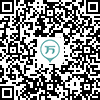120:002017年成人高考（高起点）《数学》（理工农医类）真题

1
(单项选择题)

• A.

{2，4}

• B.

{2，4，6}

• C.

{1，3，5}

• D.

{1，2，3，4，5，6}

• A
• B
• C
• D

2
(单项选择题)

• A.

• B.

• C.

• D.• A
• B
• C
• D

3
(单项选择题)

• A.• B.• C.• D.• A
• B
• C
• D

4
(单项选择题)为实数，且，则（　）.

• A.• B.• C.• D.• A
• B
• C
• D

5
(单项选择题)，且（　）.

• A.• B.• C.• D.• A
• B
• C
• D

6
(单项选择题)

• A.

1

• B.

2

• C.

6

• D.

3

• A
• B
• C
• D

7
(单项选择题)• A.

b>0，c>0

• B.

b>0，c<0

• C.

b<0，c>0

• D.

b<0，c<0

• A
• B
• C
• D

8
(单项选择题)

• A.• B.• C.• D.• A
• B
• C
• D

9
(单项选择题)

• A.

奇函数，且在(0，+∞)单调递增

• B.

偶函数，且在(0，+∞)单调递减

• C.

奇函数，且在(－∞，0)单调递减

• D.

偶函数，且在(－∞，0)单调递增

• A
• B
• C
• D

10
(单项选择题)

• A.

60个

• B.

15个

• C.

5个

• D.

10个

• A
• B
• C
• D

11
(单项选择题)，则（　）.

• A.

5m

• B.

1－m

• C.

2m

• D.

m+1

• A
• B
• C
• D

12
(单项选择题)（　）.

• A.

1

• B.

3

• C.

2

• D.

6

• A
• B
• C
• D

13
(单项选择题)

• A.• B.• C.• D.• A
• B
• C
• D

14
(单项选择题)

• A.

1

• B.

4

• C.

2

• D.• A
• B
• C
• D

15
(单项选择题)

• A.

10

• B.

20

• C.

16

• D.

26

• A
• B
• C
• D

16
(单项选择题)

• A.

100

• B.

40

• C.

10

• D.

20

• A
• B
• C
• D

17
(单项选择题)

• A.• B.• C.• D.• A
• B
• C
• D